# OliNo

Renewable Energy

## Line Lite International BV Led TL 60 cm CW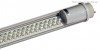presents an LED TL of 60 cm length. This article gives the measurement results. Many parameters are also found in the Eulumdat file.

See this overview for a comparison with other light bulbs.

### Summary measurement data

parameter meas. result remark
Color temperature 5843 K Cold white
Luminous intensity Iv 604 Cd
Beam angle 66 deg
Power P 8.7 W
Power Factor 0.61 For every 1 kWh net power consumed, there has been 1.3 kVAhr for reactive power..
Luminous flux 742 lm
Luminous efficacy 85 lm/W
CRI_Ra 79 Color Rendering Index.
Coordinates chromaticity diagram x=0.3253 and y=0.3333
Fitting FL-tube Direct connection to the mains voltage.
D x L external dimensions 33 x 590 mm External dimensions of the light bulb.
W x L luminous area 22 x 482 mm Dimensions of the luminous area (used in Eulumdat file). This is equal to the surface of the area on which the leds are mounted.
General remarks The ambient temperature during the whole set of measurements was 21.5 deg C.

Warm up effect: during the warm up time the  variations on illuminance and consumed power were less than 5 % and herewith negligible.

Voltage dependency: the power consumption nor illuminance are not significantly dependent on variation of the input voltage.

An additional close up photo can be found at the end of this article.

Measurement report (PDF)Eulumdat fileRight click on icon and save the file.

### Eulumdat light diagram

An interesting graph is the light diagram, indicating the intensity in the C0-C180 and the C90-C270 plane. This light diagram below comes from the program Qlumedit, that extracts these diagrams from an Eulumdat file.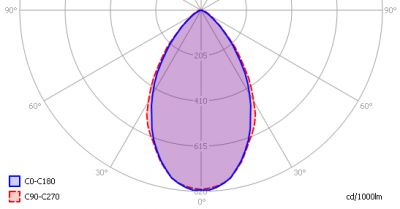The light diagram giving the radiation pattern.

The C0-C180 plane goes along the length of the tube. The C90-C270 perpendicular to that.

The light diagram indicates the luminous intensity around the light bulb. There is no significant difference between the two planes.

The unit is Cd/1000lm, meaning the intensity in Cd assuming there would be 1000 lumen in the measured light bulb. This enables comparing different types of light bulbs.

### Illuminance Ev at 1 m distance, or luminous intensity Iv

Herewith the plot of the averaged luminous intensity Iv as a function of the inclination angle with the light bulb.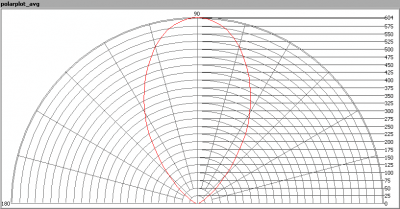The radiation pattern of the light bulb.

This radiation pattern is the average of the light output of the light diagram given earlier. Also, in this graph the luminous intensity is given in Cd.

These averaged values are used (later) to compute the lumen output.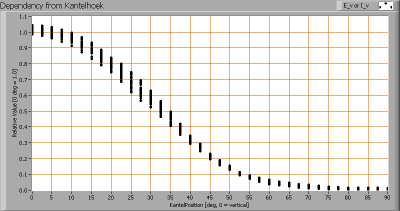Intensity data of every measured turn angle at each inclination angle.

This plot shows per inclination angle the intensity measurement results for each turn angle at that inclination angle. There are small differences in illuminance values for different turn angles. However for further calculations the averaged values will be used.

When using the average values per inclination angle, the beam angle can be computed, being 66 degrees. This is the average value for all C-planes on average. There is little difference between the C-planes so this beam angle is accurate for most C-planes.

### Luminous flux

With the averaged illuminance data at 1 m distance, taken from the graph showing the averaged radiation pattern, it is possible to compute the luminous flux.

The result of this computation for this light spot is a luminous flux of 742 lm.

### Luminous efficacy

The luminous flux being 742, and the power of the light bulb 8.7 W, yields a luminous efficacy of 85 lm/W.

A power factor of 0.61 means that for every 1 kWh net power consumed, a reactive component of 1.3 kVAr was needed.

 Light bulb voltage 230 VAC Light bulb current 62 mA Power P 8.7 W Apparent power S 14.1 VA Power factor 0.61

Of this light bulb the voltage across ad the resulting current through it are measured and graphed.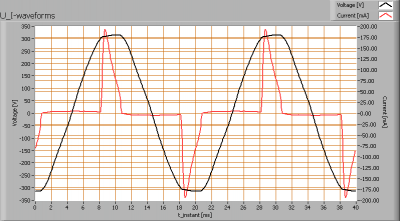Voltage across and current through the light bulb

There are peaks in current just before the voltage hits its maximum value. This results in a waveform for the current that is different from the sine form of the voltage, resulting in a power factor less than 1. The peak is also not symmetrical, resulting in a further decrease of the power factor.

Also the power spectrum of the current is determined.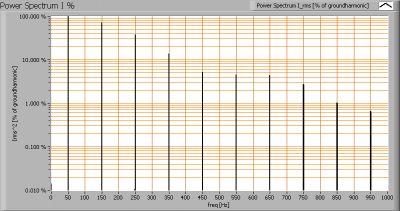Current power spectrum in % of the first harmonic (50 Hz).

The current peaks result in a current power spectrum with a considerable amount of higher harmonics.

### Color temperature and Spectral power distribution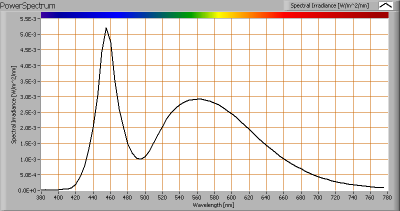The spectral power distribution of this light bulb.

The measured color temperature is about 5850 K which is cold white.

This color temperature is measured straight underneath the light bulb. Below a graph showing the color temperature for different inclination angles.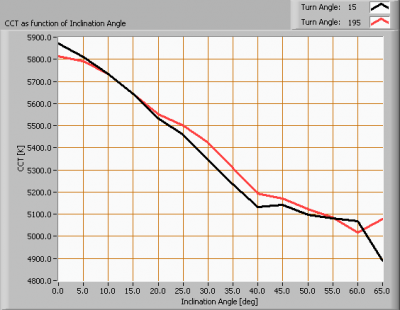Color temperature as a function of inclination angle.

The color temperature is given for inclination angles up to 65 degrees, since at higher angles there is almost no light output anymore.

The value drops about 14 %.

### Chromaticity diagram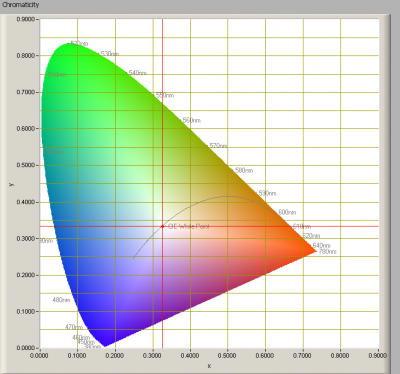The chromaticity space and the position of the lamp’s color coordinates in it.

The light coming from this lamp on top of the Planckian Locus (the black path in the graph).

Its coordinates are x=0.3253 and y=0.3333.

### Color Rendering Index (CRI) or also Ra

Herewith the image showing the CRI as well as how well different colors are represented (rendered). The higher the number, the better the resemblance with the color when a black body radiator would have been used (the sun, or an incandescent lamp).

Each color has an index Rx, and the first 8 indexes (R1 .. R8) are averaged to compute the Ra which is equivalent to the CRI.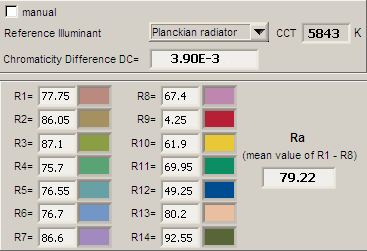CRI of the light of this lightbulb.

The value of 79 is a little lower than 80 which is considered a minimum value for indoor usage.

Note: the chromaticity difference is 0.0039 indicates the distance to the Planckian Locus. Its value is lower than 0.0054, which means that the calculated CRI result is meaningful.

### Voltage dependency

The dependency of a number of lamp parameters on the lamp voltage is determined. For this, the lamp voltage has been varied and its effect on the following light bulb parameters measured: illuminance E_v [lx], the lamppower P [W], the (Correlated) Color Temperature [K] and the luminous efficacy [lm/W].Lamp voltage dependencies of certain light bulb parameters, where the value at 230 V is taken as 100 %.

The consumed power nor illuminance vary insignificantly (< 5 %) when the voltage is varied between 200-250 V.

When the voltage at 230 V varies with + and – 5 V, then the illuminance varies within 0.5 %, so when abrupt voltage changes occur this effect is not visible in the illuminance output.

### Warm up effects

After switch on of a cold lamp, the effect of heating up of the lamp is measured on illuminance E_v [lx], color temperature CT or correlated color temperature CCT [K], the lamppower P [W] and the luminous efficacy [lm/W].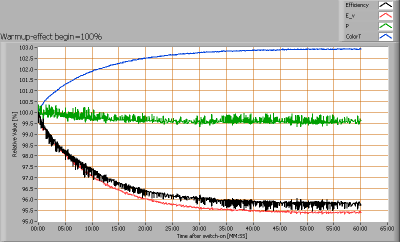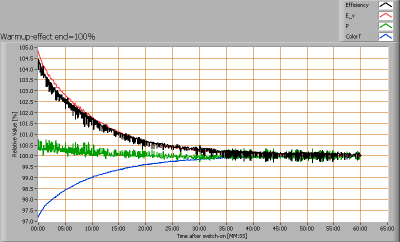Effect of warming up on different light bulb parameters. At top the 100 % level is put at begin, and at bottom at the end.

The variations of the illuminance and consumed power are less than 5 %, herewith negligible.